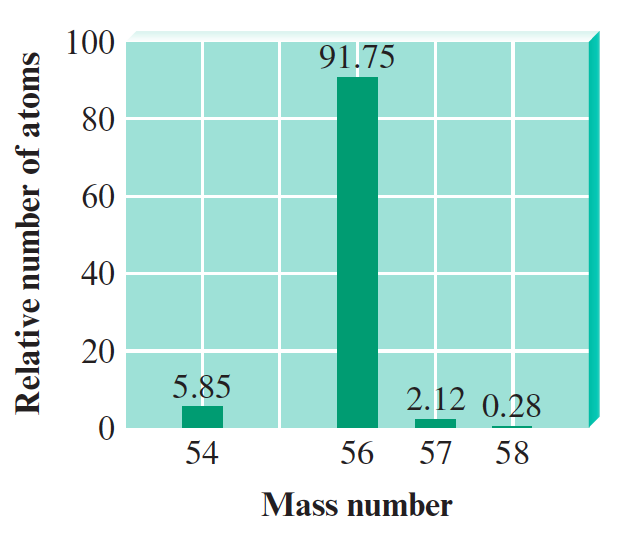# Problem: The stable isotopes of iron are 54Fe, 56Fe, 57Fe, and 58Fe. The mass spectrum of iron looks like the following:Use the data on the mass spectrum to estimate the average atomic mass of iron, and compare it to the value given in the periodic table.

###### FREE Expert Solution
95% (163 ratings)
###### FREE Expert Solution

We can use the following equation:

where:

atomic mass = average atomic mass of the element

f.a. = fractional abundance of the isotope

To get f.a., we simply need to divide the given percent abundance by 100.

95% (163 ratings)###### Problem Details

The stable isotopes of iron are 54Fe, 56Fe, 57Fe, and 58Fe. The mass spectrum of iron looks like the following:Use the data on the mass spectrum to estimate the average atomic mass of iron, and compare it to the value given in the periodic table.

Frequently Asked Questions

What scientific concept do you need to know in order to solve this problem?

Our tutors have indicated that to solve this problem you will need to apply the Mass Spectrometry concept. If you need more Mass Spectrometry practice, you can also practice Mass Spectrometry practice problems.

What professor is this problem relevant for?

Based on our data, we think this problem is relevant for Professor Norton's class at UGA.

What textbook is this problem found in?

Our data indicates that this problem or a close variation was asked in Chemistry: An Atoms First Approach - Zumdahl Atoms 1st 2nd Edition. You can also practice Chemistry: An Atoms First Approach - Zumdahl Atoms 1st 2nd Edition practice problems.